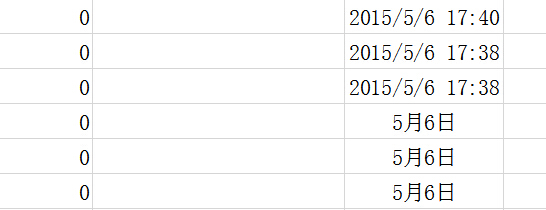2015-05-06 08:49

# 用bva让一个单元格显示另一个单元格的修改日期，且能决定日期显示的格式

Private Sub Worksheet_Change(ByVal Target As Range)
If Target.Count = 1 Then
If Target.Column = 4 Then
Cells(Target.Row, 6) = Now
End If
End If
End Sub

• 点赞
• 写回答
• 关注问题
• 收藏
• 复制链接分享
• 邀请回答

#### 3条回答

• `````` Private Sub Worksheet_Change(ByVal Target As Range)
If Target.Count = 1 Then
If Target.Column = 4 Then
Cells(Target.Row, 6).NumberFormatLocal = "yyyy-m-d" '年月日
'Cells(Target.Row, 6).NumberFormatLocal = "m-d" '月日
Cells(Target.Row, 6) = Now
End If
End If
End Sub
``````
点赞 评论 复制链接分享
•我想要的是 就像下半部那样出现时间，而不是像上半部那样

点赞 评论 复制链接分享
• 点赞 评论 复制链接分享